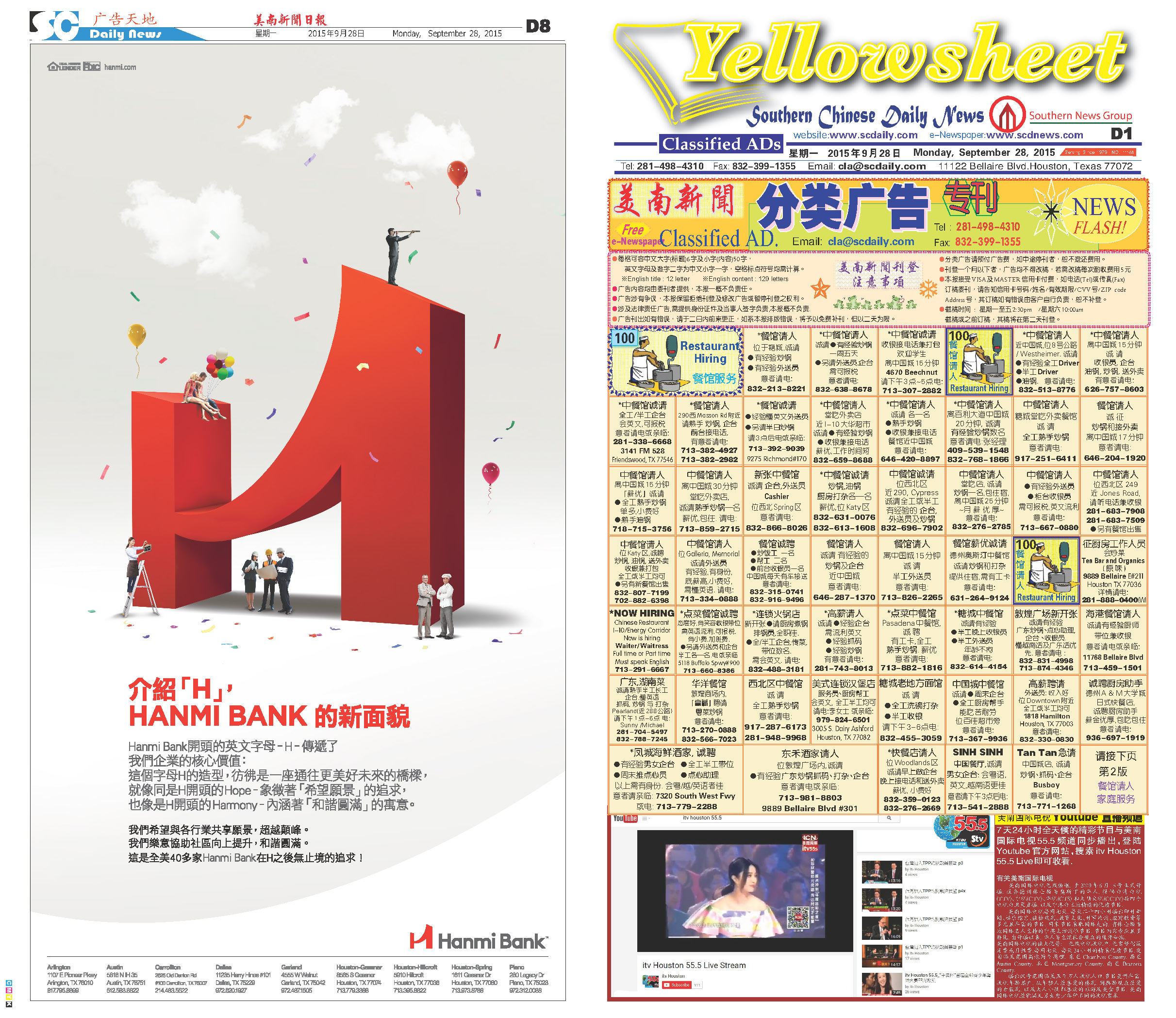151007 Epaper

 A Section B SectionC Section D Section151006 Epaper

 A Section B SectionC Section D Section151005 Epaper

 A Section B SectionC Section D Section151004 Epaper

 A Section B SectionC Section D Section151003 Epaper

 A Section B SectionC Section D Section151002 Epaper

 A Section B SectionC Section D Section151001 Epaper

 A Section B SectionC Section D Section150930 Epaper

 A Section B SectionC Section D Section150928 Epaper

 A Section B SectionC Section D Section150929 Epaper

 A Section B SectionC Section D Section150927 Epaper

 A Section B SectionC Section D Section